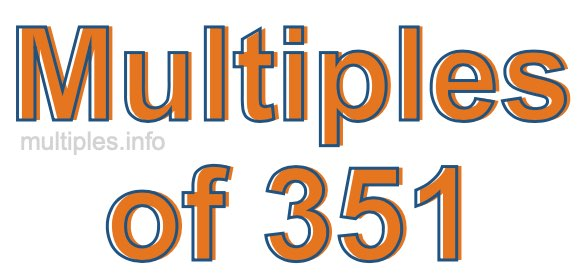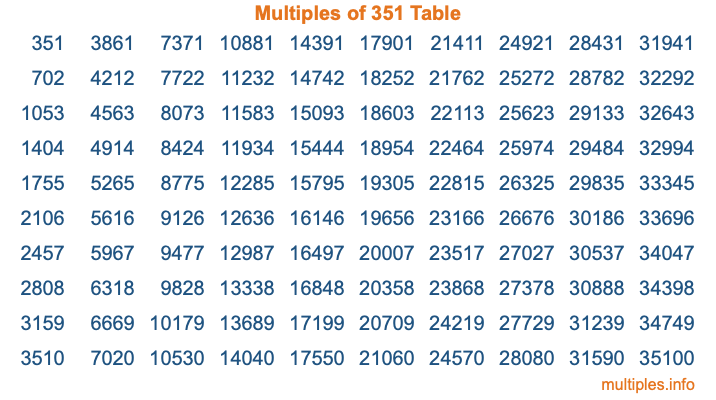Multiples of 351Welcome to the Multiples of 351 page. Here we will first teach you everything you will ever need to know about the multiples of 351, and then give you a study guide summary of everything we taught you to make sure you remember it all. Use this page to look up facts and learn information about the multiples of 351. This page will make you a multiples of three hundred fifty-one expert!

Definition of Multiples of 351
Multiples of 351 are all the numbers that when divided by 351 equal an integer. Each of the multiples of 351 are called a multiple. A multiple of 351 is created by multiplying 351 by an integer.

Therefore, to create a list of multiples of 351, you start with 1 multiplied by 351, then 2 multiplied by 351, then 3 multiplied by 351, and so on for as long as you want. Thus, the list of the first five multiples of 351 is 351, 702, 1053, 1404, and 1755. To see a larger list of multiples of 351, see the printable image of Multiples of 351 further down on this page. We also have a category where you can choose any nth multiple of 351.

Multiples of 351 Checker
The Multiples of 351 Checker below checks to see if any number of your choice is a multiple of 351. In other words, it checks to see if there is any number (integer) that when multiplied by 351 will equal your number. To do that, we divide your number by 351. If the the quotient is an integer, then your number is a multiple of 351.

Is  a multiple of 351?

Least Common Multiple of 351 and ...
A Least Common Multiple (LCM) is the lowest multiple that two or more numbers have in common. This is also called the smallest common multiple or lowest common multiple and is useful to know when you are adding our subtracting fractions. Enter one or more numbers below (351 is already entered) to find the LCM.

Check out our LCM Calculator if you need more details about the Least Common Multiple or if you need the LCM for different numbers for adding and subtraction fractions.

nth Multiple of 351
As we stated above, 351 is the first multiple of 351, 702 is the second multiple of 351, 1053 is the third multiple of 351, and so on. Enter a number below to find the nth multiple of 351.

th multiple of 351

Multiples of 351 vs Factors of 351
351 is a multiple of 351 and a factor of 351, but that is where the similarities end. All postive multiples of 351 are 351 or greater than 351. All positive factors of 351 are 351 or less than 351.

Below is the beginning list of multiples of 351 and the factors of 351 so you can compare:

Multiples of 351: 351, 702, 1053, 1404, 1755, etc.

Factors of 351: 1, 3, 9, 13, 27, 39, 117, 351

As you can see, the multiples of 351 are all the numbers that you can divide by 351 to get a whole number. The factors of 351, on the other hand, are all the whole numbers that you can multiply by another whole number to get 351.

It's also interesting to note that if a number (x) is a factor of 351, then 351 will also be a multiple of that number (x).

Multiples of 351 vs Divisors of 351
The divisors of 351 are all the integers that 351 can be divided by evenly. Below is a list of the divisors of 351.

Divisors of 351: 1, 3, 9, 13, 27, 39, 117, 351

The interesting thing to note here is that if you take any multiple of 351 and divide it by a divisor of 351, you will see that the quotient is an integer.

Multiples of 351 Table
Below is an image of the first 100 multiples of 351 in a table. The table is in chronological order, column by column. The first column has the first ten multiples of 351, the second column has the next ten multiples of 351, and so on.The Multiples of 351 Table is also referred to as the 351 Times Table or Times Table of 351. You are welcome to print out our table for your studies.

Negative Multiples of 351
Although not often discussed or needed in math, it is worth mentioning that you can make a list of negative multiples of 351 by multiplying 351 by -1, then by -2, then by -3, and so on, to get the following list of negative multiples of 351:

-351, -702, -1053, -1404, -1755, etc.

Multiples of 351 Summary
Below is a summary of important Multiples of 351 facts that we have discussed on this page. To retain the knowledge on this page, we recommend that you read through the summary and explain to yourself or a study partner why they hold true.

There are an infinite number of multiples of 351.

A multiple of 351 divided by 351 will equal a whole number.

351 divided by a factor of 351 equals a divisor of 351.

The nth multiple of 351 is n times 351.

The largest factor of 351 is equal to the first positive multiple of 351.

351 is a multiple of every factor of 351.

351 is a multiple of 351.

A multiple of 351 divided by a divisor of 351 equals an integer.

351 divided by a divisor of 351 equals a factor of 351.

Any integer times 351 will equal a multiple of 351.

Multiples of a Number
Here you can get the multiples of another number, all with the same attention to detail as we did for multiples of 351 on this page.

Multiples of
Multiples of 352
Did you find our page about multiples of three hundred fifty-one educational? Do you want more knowledge? Check out the multiples of the next number on our list!